## Solving Equations

### Solving Equations You Never Thought Possible

Well, well, well. Look who's back for more. So you think you have the basics down, do you? You think that you've mastered simplifying radicals? What about fractional and negative exponents? That may be true, but you haven't really mastered this chapter until you've mastered solving for a missing variable. This is truly the whole fly-in-the-hand experience you absolutely need to have.

For better or worse, we're going to assume that you already have the basics of solving algebraic equations down. We go to bed at night hoping that you know how to add, subtract, multiply, and divide your way to solving for x. However, the one thing you may or may not have seen before is how to undo a square or square root in order to get little ol' x all by his lonesome.

We're going to take the liberty of skipping right to a few sample problems. We think you'll get the hang of it pretty quickly.

Now go catch some flies.

### Sample Problem

Solve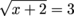for x.

Ultimately, our goal in any solving situation is to get the variable by itself. To do so, we want to undo every operation that's been done to x. The first thing to undo is the square root, so we'll use the inverse operation of the square root. In other words, square both sides.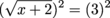x + 2 = 9

This makes things pretty easy to manage. Let's go ahead and undo our addition by subtracting 2 from both sides.

x + 2 = 9
x = 7

Fly number one: check.

### Sample Problem

Solve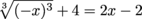for x.

Once again, we're faced with the task of getting x by itself. We'll start by simplifying that crazy radically exponential thingy-ma-bob on the left. It will also answer to its other name: a term. To do this, we need to take the third root of (-x)3. Since these are inverse operations of each other, we have…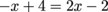Nice. The cube root cancels out the exponent. From here, it's pretty basic algebra. We'll finish things up by adding x and 2 to both sides.

-x + 4 = 2x – 2
6 = 3x

Finally, to undo our multiplication, we can divide both sides by 3. This gives us our final answer.

6 = 3x
2 = x

### Sample Problem

Solve: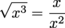.

Now we're talking. Our first step has got to be to simplify this thing. The question is: how? All we do is rewrite the left side using fractional exponents. For the right side, we'll use our exponent properties but keep things positive. After that, we'll evaluate our situation.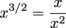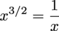Multiplying both sides by x here seems like the way to go. However, because this means that x is no longer in the denominator, it's important to note that no matter where our work takes us from here, x cannot equal 0.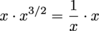x1 + 3/2 = 1
x2/2 + 3/2 = 1
x5/2 = 1

The equation we have now can be written in two ways: x5/2 = 1 or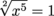. We're fans of going back to the non-fractional version in order to finish things up. This allows us to easily see that our next step will be to square both sides so we can get rid of that pesky square root.

x5/2 = 1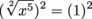x5 = 1

Finally, we can undo the exponent by taking the fifth root of both sides.

x5 = 1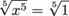x = 1

All in all, this problem worked out extremely well, since 12 is 1 and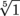is also just 1. Life may not always be so kind.

### Sample Problem

Solve: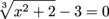.

Once again, we need to solve for x. However, it's got some serious math-armor: there are a ton of different operations protecting it from being by itself. Time to chisel away at them one by one. To start, we'll add 3 to both sides.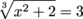Next, we cancel out the cube roots by cubing both sides.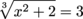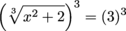x2 + 2 = 27

To undo the addition of 2, we'll subtract 2 from both sides. No surprise there.

x2 + 2 = 27
x2 = 25

Finally, we can take the square root of both sides in order to find our answer. Here's the deal, though: every positive real number actually has two square roots. While we'll get into exactly what a real number is a little bit later, for now we'll say this:

x2 = 25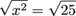x = ±5

This ± symbol is called a "plus-or-minus sign" and simply means we have two solutions, +5 or -5.

### Sample Problem

Solve: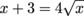.

This problem looks simple enough. Right? Since we can't combine any like terms here, we wanna get rid of that pesky square root. To do this, we have no choice but to square both sides.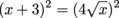(x + 3)2 = 16x

Great. Things didn't look too complicated before, but now there's a binomial on the left. Yikes. At least we don't have any square roots left. At this point, the number one thing young noobs might do is to just sit there and stare. But there has to be something to do. Why not multiply out the binomial?

(x + 3)2 = 16x
(x + 3)(x + 3) = 16x
x
2 + 6x + 9 = 16x

Things still aren't peachy right about now. But you have to admit we're getting closer. The last time we had a quadratic, the best way to solve was to set things equal to 0. The same idea applies here. Let's subtract 16x from both sides.

x2 + 6x + 9 = 16x
x2 – 10x + 9 = 0

Next, unless we can get this thing to factor, we're going to have to pull out the quadratic formula. See what we mean about this being the fly-catching section? Lucky for us, the quadratic factors ever so nicely.

x2 – 10x + 9 = 0
(x – 1)(x – 9) = 0

Finally, we know that if two things have a product of 0, one of them just has to be 0. There's no choice. To solve, we'll set each factor equal to 0. This will give us two solutions:

(x – 9) = 0
x = 9

Or:

(x – 1) = 0
x = 1

Boom. Roasted.